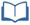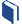# Situation Simulated

Estimated Time: 180 minutes

###Lesson Objectives:

• The students will design, conduct and interpret the results of a simulation.
• The students will also use simulation models for random sampling to develop margin of error and decide if difference in parameters are significant.

###Resources for this lesson:

Before you begin, download your Algebra II Journalfor Situation Simulated. You will be completing activities in the journal throughout this lesson.

Visit this section on each page to access information about key terms and other resources to help facilitate the lesson.

> Glossary> Calculator Resources> Teacher Resources: Instructional NotesKey Common Core State Standards:

S.IC.2: Decide if a specified model is consistent with results from a given data-generating process, e.g., using simulation. For example, a model says a spinning coin falls heads up with probability 0.5. Would a result of 5 tails in a row cause you to question the model? Make inferences and justify conclusions from sample surveys, experiments, and observational studies

S.IC.4: Use data from a sample survey to estimate a population mean or proportion; develop a margin of error through the use of simulation models for random sampling.

S.IC.5: Use data from a randomized experiment to compare two treatments; use simulations to decide if differences between parameters are significant

Supporting Common Core State Standards:
N/A

Standards for Mathematical Practice Emphasized:

1. Make sense of problems and persevere in solving them.
2. Reason abstractly and quantitatively.
3. Construct viable arguments and critique the reasoning of others.
4. Model with mathematics.
5. Use appropriate tools strategically.
7. Look for and make use of structure.
8. Look for and express regularity in repeated reasoning.

Next >